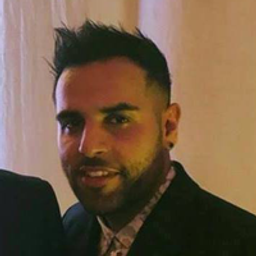# Geometry

## Educators### Problem 1

Suppose $\triangle B I G \cong \triangle C A T$ . Complete.
$$\angle G \cong \underline{?}$$Amrita B.

### Problem 2

Suppose $\triangle B I G \cong \triangle C A T$ . Complete.
$$\underline{?}=m \angle A$$Kashif Q.

### Problem 3

Suppose $\triangle B I G \cong \triangle C A T$ . Complete.
$$B I=?$$Amrita B.

### Problem 4

Suppose $\triangle B I G \cong \triangle C A T$ . Complete.
$$\stackrel{?}{\longrightarrow} \cong \overline{A T}$$Kashif Q.

### Problem 5

Suppose $\triangle B I G \cong \triangle C A T$ . Complete.
$$\triangle I G B \cong \stackrel{?}{\longrightarrow}$$Amrita B.

### Problem 6

Suppose $\triangle B I G \cong \triangle C A T$ . Complete.
$$\underline{?} \cong \triangle C T A$$Kashif Q.

### Problem 7

If $\triangle D E F \cong \triangle R S T, m \angle D=100,$ and $m \angle F=40$ , name four congruent angles.Amrita B.

### Problem 8

Is the statement "Corresponding parts of congruent triangles are congruent" based on a definition, postulate, or theorem?Kashif Q.

### Problem 9

Suppose $\triangle L X R \cong \triangle F N E$ . List six congruences that can be justified by the following reason: Corr. parts of $\cong \triangle$ are $\cong .$Amrita B.

### Problem 10

The two triangles shown are congruent. Complete.
a. $\triangle S T O \cong$
b. $\angle S \cong \underline{?}$ because $\underline{\text { ? }}$
c. $\overline{S O} \cong \underline{?}$ because-? Then point $O$ is the midpoint of $\underline{\text { . }}$
d. $\angle T \cong \frac{?}{S T} \| \overline{R K}$ because Then $\overline{S T} \| \overline{R K}$ becauseKashif Q.

### Problem 11

The two triangles shown are congruent. Complete.
a. $\triangle P A L \cong \stackrel{?}{\longrightarrow}$
b. $\overline{P A} \cong \longrightarrow$
c. $\angle 1 \cong \underline{?}$ because $\underline{\longrightarrow}$ Then $\overline{P A} \| \underline{?}$ because $\underline{?}$
d. $\angle 2 \cong \stackrel{?}{\text { because }}$ Then $\underline{?} \| \underline{?}$ because $\underline{?}$Amrita B.

### Problem 12

Plot the given points on graph paper. Draw $\triangle F A T$ . Locate point $C$ so that
$$F(1,2) \quad A(4,7) \quad T(4,2)$$Kashif Q.

### Problem 13

Plot the given points on graph paper. Draw $\triangle F A T$ . Locate point $C$ so that
$$F(7,5) \qquad A(-2,2) \qquad T(5,2)$$Amrita B.

### Problem 14

Plot the given points on graph paper. Draw $\triangle A B C$ and $\triangle D E F$ . Copy and complete the statement $\triangle A B C \cong \longrightarrow$ .
$$\begin{array}{lll}{A(-1,2)} & {B(4,2)} & {C(2,4)} \\ {D(5,-1)} & {E(7,1)} & {F(10,-1)}\end{array}$$Kashif Q.

### Problem 15

Plot the given points on graph paper. Draw $\triangle A B C$ and $\triangle D E F$ . Copy and complete the statement $\triangle A B C \cong \longrightarrow$ .
$$\begin{array}{lll}{A(-7,-3)} & {B(-2,-3)} & {C(-2,0)} \\ {D(0,1)} & {E(5,1)} & {F(0,-2)}\end{array}$$Amrita B.

### Problem 16

Plot the given points on graph paper. Draw $\triangle A B C$ and $\triangle D E F$ . Copy and complete the statement $\triangle A B C \cong \longrightarrow$ .
$$\begin{array}{lll}{A(-3,1)} & {B(2,1)} & {C(2,3)} \\ {D(4,3)} & {E(6,3)} & {F(6,8)}\end{array}$$

Check back soon!

### Problem 17

Plot the given points on graph paper. Draw $\triangle A B C$ and $\triangle D E F$ . Copy and complete the statement $\triangle A B C \cong \longrightarrow$ .
$$\begin{array}{lll}{A(1,1)} & {B(8,1)} & {C(4,3)} \\ {D(3,-7)} & {E(5,-3)} & {F(3,0)}\end{array}$$Amrita B.

### Problem 18

Plot the given points on graph paper. Draw $\triangle A B C$ and $\overline{D E} .$ Find two locations of point $F$ such that $\triangle A B C \cong \triangle D E F .$
$$A(1,2) \qquad B(4,2) \qquad C(2,4) \qquad D(6,4) \quad E(6,7)$$

Check back soon!

### Problem 19

Plot the given points on graph paper. Draw $\triangle A B C$ and $\overline{D E} .$ Find two locations of point $F$ such that $\triangle A B C \cong \triangle D E F .$
$$A(-1,0) \qquad B(-5,4) \qquad C(-6,1) \qquad D(1,0) \quad E(5,4)$$Amrita B.

### Problem 20

Complete: quad. $N E R O \cong$ quad.

Check back soon!

### Problem 21

In your own words explain why each of the following statements must be true.
a. $O$ is the midpoint of $\overline{N M}$ .
b. $\angle N O R \cong \angle M O R$
c. $\frac{\angle N O R \cong \angle M O R}{N M}$Amrita B.

### Problem 22

Accurately draw each triangle described. Predict whether your triangle will be congruent to your classmates.
$$\begin{array}{l}{\text { a. In } \triangle R S T, R S=4 \mathrm{cm}, m \angle S=45, \text { and } S T=6 \mathrm{cm} .} \\ {\text { b. In } \triangle U V W, m \angle U=30, U V=5 \mathrm{cm}, \text { and } m \angle V=100} \\ {\text { c. In } \triangle D E F, m \angle D=30, m \angle E=68, \text { and } m \angle F=82}\end{array}$$
d. In $\Delta X Y Z, \quad X Y=3 \mathrm{cm}, \quad Y Z=5 \mathrm{cm},$ and $X Z=6 \mathrm{cm} .$ (Try for a reasonably accurate drawing. You may find it helpful to cut a thin strip of paper for each side. then form the triangle.)

Check back soon!

### Problem 23

Does congruence of triangles have the reflexive property? the symmetric property? the transitive property?Amrita B.
Suppose you are given a scalene triangle and a point $P$ on some line $l$ . How many triangles are there with one vertex at $P$ , another vertex on $l$ . and each triangle congruent to the given triangle?